User:Tohline/Appendix/CGH/Overview

(Difference between revisions)
 Revision as of 21:25, 27 December 2017 (view source)Tohline (Talk | contribs) (→CGH: Philosophical Overview)← Older edit Revision as of 21:47, 27 December 2017 (view source)Tohline (Talk | contribs) (→Single Aperture)Newer edit → Line 71: Line 71: [Z^2 + y_1^2  ]^{1 / 2} \, . [Z^2 + y_1^2  ]^{1 / 2} \, . [/itex] [/itex] + + + + + Notice that, in the definition of $~D_j$, the expression inside the square brackets involves a term that depends quadratically on the dimensionless length scale, $~Y_j/L$, as well as a term that depends linearly on this ratio.  As discussed below, our expression for the amplitude simplifies nicely in situations where the quadratic term can be ignored.  Via a related simplification, we will find that the various natural lengths of this problem are can be related via the expression, +
+ + +
+ $~y_1\biggr|_{1^\mathrm{st} \mathrm{fringe}}$ + + $~\approx$ + + $~\frac{\lambda Z}{w} \, .$
+ + +

CGH: Philosophical Overview

Slit Diffraction

Single Aperture

As has been detailed in an accompanying discussion, we consider, first, the amplitude (and phase) of light that is incident at a location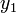$~y_1$ on an image screen that is located a distance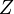$~Z$ from a slit of width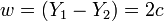$~w = (Y_1 - Y_2) = 2c$. The amplitude is given by the expression,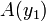$~A(y_1)$$~=$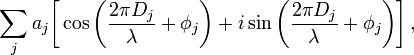$~\sum_j a_j \biggl[ \cos\biggl(\frac{2\pi D_j}{\lambda} + \phi_j \biggr) + i \sin\biggl(\frac{2\pi D_j}{\lambda} + \phi_j \biggr) \biggr] \, ,$

where,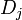$~D_j$$~=$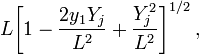$~ L \biggl[1 - \frac{2y_1 Y_j}{L^2} + \frac{Y_j^2}{L^2} \biggr]^{1 / 2} \, ,$

and,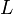$~L$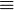$~\equiv$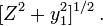$~ [Z^2 + y_1^2 ]^{1 / 2} \, .$

Notice that, in the definition of$~D_j$, the expression inside the square brackets involves a term that depends quadratically on the dimensionless length scale,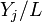$~Y_j/L$, as well as a term that depends linearly on this ratio. As discussed below, our expression for the amplitude simplifies nicely in situations where the quadratic term can be ignored. Via a related simplification, we will find that the various natural lengths of this problem are can be related via the expression,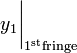$~y_1\biggr|_{1^\mathrm{st} \mathrm{fringe}}$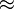$~\approx$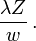$~\frac{\lambda Z}{w} \, .$